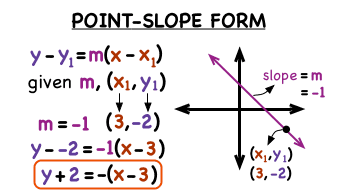# Question #f10f7

Oct 27, 2017

Slope-intercept form is $y = m x + b$.

#### Explanation:

In slope-intercept form

$y = m \left(\text{slope")x+b("y"-"intercept}\right)$There is also standard form:

$A y + B x = C$

where $A$, $B$, and $C$ are numbers and $x$ and $y$ are your variables.The final form is point-slope form:

$y - {y}_{1} = m \left(x - {x}_{1}\right)$

Here $m$ will equal your slope and $x$ and $y$ are going to be your variables. The ${x}_{1}$ and ${y}_{1}$, however, are going to be the coordinates of a point on your lineThanks to virtualnerd.com !!